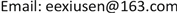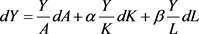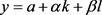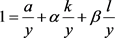﻿ 索洛余值结合多元回归对我国快递行业经济增长因素的实证分析 An Empirical Analysis of Solow Residual Value Combined with Multivariate Regression on the Factors of Economic Growth in China’s Logistics Industry

Modern Marketing
Vol. 09  No. 03 ( 2019 ), Article ID: 31524 , 5 pages
10.12677/MOM.2019.93003

An Empirical Analysis of Solow Residual Value Combined with Multivariate Regression on the Factors of Economic Growth in China’s Logistics Industry

Mengjie Hou, Xiusen Li

School of Mathematics and Statistics, Shandong University of Technology, Zibo ShandongReceived: Jul. 10th, 2019; accepted: Jul. 23rd, 2019; published: Jul. 30th, 2019ABSTRACT

We discuss the economic growth of express industry. According to Cobb Douglas production function, this paper uses SPSS to carry out multiple regression analysis, calculates the contribution rate of each factor input to the total output of express industry by Solow Remainder Method, and then introduces various factors to establish economic growth model, so as to analyze the economic growth factors of the total output of express industry. The results are quite in line with the reality.

Keywords:Express Industry, Solow Model, C-D Production Function, Multivariate Regression Analysis1. 引言

2. 经济增长因素指标的建立

2.1. Cobb-Douglas生产函数

Cobb-Douglas生产函数，由希克斯中性生产函数经过微分以及积分的变换，得生产函数： $Y=A{K}^{\alpha }{L}^{\beta }$ 为幂形式，其中Y为产出量、K为资本、L为劳动投入量；A表示技术进步水平；α表示资本对产出的

2.2. 索洛余值

$\frac{dY}{Y}=\frac{dA}{A}+\alpha \frac{dK}{K}+\beta \frac{dL}{L}$

a. 在把Cobb-Douglas生产函数 $Y=A{K}^{\alpha }{L}^{\beta }$ 式进行微分后两边同除以Y得到：$\frac{\Delta Y}{Y}=\frac{\Delta A}{A}+\alpha \frac{\Delta K}{K}+\beta \frac{\Delta L}{L}$

b. 可以采用水平法(几何法)计算平均增长率y，k，l。式两边同时除以y得到，这就可以得出技术增长对产出增长的贡献率---全要素生产率 $\frac{a}{y}$ ，即除劳动力和金融对产出的贡献外，所有因素对产出的贡献率。通常总结为技术进步的贡献，视为索洛余值。而资本的增加和劳动力增长对产出增长所做的贡献率分别为： $\alpha \frac{k}{y}\text{}；\text{}\beta \frac{l}{y}$

3. 实证分析

a. 因变量：lny；b. 预测变量：(常量)，lnx2。Table 2. Economic growth analysis table of express industry in China

a. 因变量：lny；b. 预测变量：(常量)，lnx1；c. 预测变量：(常量)，lnx1，lnx4。Table 4. Economic growth analysis table of China’s multi-factor express industry

4. 结论和意义

An Empirical Analysis of Solow Residual Value Combined with Multivariate Regression on the Factors of Economic Growth in China’s Logistics Industry[J]. 现代市场营销, 2019, 09(03): 17-21. https://doi.org/10.12677/MOM.2019.93003

1. 1. 罗伯特•索洛. 经济增长因素分析[M]. 北京: 商务印书馆, 1999.

2. 2. 毛健. 经济增长理论探索[M]. 北京: 商务印书馆, 2009.

3. 3. 薛薇. 统计分析与SPSS的应用[M]. 北京: 中国人民大学出版社, 2007.

4. 4. Griliches, Z. (1979) Issues in Assessing the Contribution of Research and Development to Productivity Growth. Bell Journal of Economics, 10, 92-116.